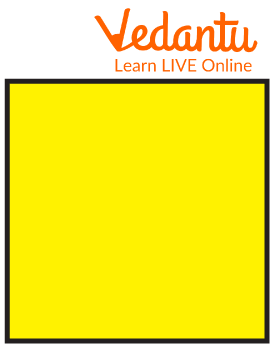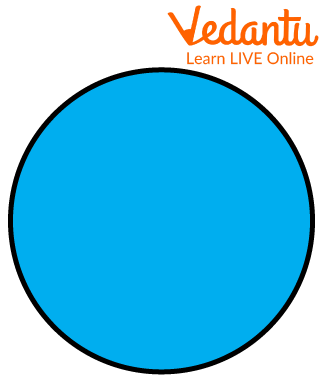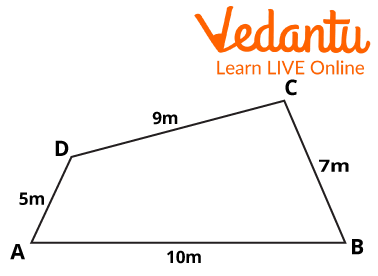Courses
Courses for Kids
Free study material
Offline Centres
More

# Perimeter of a FigureLast updated date: 27th Nov 2023
Total views: 99.3k
Views today: 0.99k## Introduction to Perimeter of a Figure

The route or boundary that encircles any shape in mathematics is defined by the shape's circumference. To put it another way, the circumference and perimeter both refer to the length of a shape's outline.

## What is the Perimeter?

A path that encircles a two-dimensional object is called a perimeter. The word is Greek, peri meaning "around," and "metre" meaning measure. The phrase can refer to either the path or its length; it can be compared to the size of a shape's outline.

## How to Find the Perimeter of a Figure?

Steps to find the Perimeter of a given figure:

STEP 1 : Calculate the lengths of the sides.

Usually we don't need a special formula to get perimeter because perimeter is simply a measure of a two-dimensional figure's outline (though there are equations for specific shapes to make it easier).

STEP 2 : The length of each side is added together.

When calculating the perimeter of a non-circular object, add up all of the side lengths to get its perimeter.

STEP 3 : Be careful of the units of measurement.

We need to add all the lengths in the same unit to add else we can't add it (such as feet, miles, or metres).

## Perimeter of Square:

Since a square is a polygon with four equal sides, its perimeter will be equal to the sum of those sides.

The formula ${\rm{P = 4x}}$, where x is the length of one side, can be used to determine whether a square has four equal-length sides.Square

## Perimeter of Rectangle:

The perimeter of a rectangle is determined by adding up all of its sides.

Formula $= 2(a + b)$ units

where:

“a” is the length of the rectangle

“b” is the breadth of the rectangleRectangle

## Perimeter of Circle:

Circumference of the circle or perimeter of the circle is the measurement of the boundary of the circle.

Circle perimeter or circumference is equal to$2\pi r$ .

where,

$\Pi$ is a mathematical constant with an estimated value of 3.14 (to the nearest two decimal places).Circle

## Solved Examples:

1: Find the perimeter of the square whose side is 6 units.

Ans: As we know that all the sides of a square are equal.

And its perimeter is 4 times its side.

Thus, it’s perimeter will be $4 \times 6 = 24$

Its perimeter is 24 units.

2: Find the perimeter of the following figure:Ans: The perimeter of a planar object is the sum of all the sides in it.

It can alternatively be described as the total sum of all of a planar object's bounding sides.

Here,$AB + BC + CD + DA = 5 + 9 + 7 + 10$ is the total length of all the sides of the provided figure.

Thus, The stated figure's perimeter is 31 metres.

3 :Determine the circle's radius for$C = 50cm$ .

Ans: Given that 50 cm is the circumference.

According to the formula$C = 2\pi r$

This indicates that

$\begin{array}{l}50 = 2\pi r\\25 = \pi r\\r = 25/\pi \end{array}$

As a result, the circle's radius is $25/\pi$ cm.

## Conclusion:

Any 2D shape's perimeter can be calculated by labelling and adding the sides. When calculating the perimeter, don't forget to account for the length of each side. For instance, the lengths of a rectangle's two widthwise edges and its two lengthwise borders are added to form the rectangle's perimeter and similar for other shapes also.

## FAQs on Perimeter of a Figure

1. How can I determine the perimeter of a non-regular (irregular) shape?

The lengths of all the sides must be added together in order to determine an irregular shape's perimeter. If such lengths are not provided, you can be given alternative information that can assist you in locating those lengths.

2. Is perimeter adding or multiplying the sides together?

The perimeter is the length of the outline of a shape. To find the perimeter of a rectangle or square you have to add the lengths of all the four sides.

3. What is the circumference of a circle?

The distance along a circle's perimeter is referred to as its circumference. In other words, the circumference of a circle is equal to the length of a straight line formed by opening up the circle.

4. Determine the circumference of a circle whose radius is 5 units.

Given , $Radius = 5 units$

We know that , $C = 2\pi r$

$\begin{array}{l}C = 2 \times \pi \times 5\\C = 10\pi \end{array}$

Thus , Circumference = 10 π  units.

5. What is the importance of perimeter in our daily life?

In daily life, we frequently use the concept of perimeter.